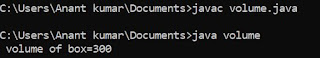## Saturday, 14 April 2018

### Program to find simple interest in C

Here we are going to write program to find simple interest. First of all we declare four variable and then input the variable of P,N,R.These are principal ,rate number of year and then use formula for simple interest. Now we are going to write the program.

#include<stdio.h>
void main ()
float p,n,r,s.i;
clrscr();
printf ("/n Enter value of p:");
scanf ("%f",& p);
printf ("/n Enter value of r:");
scanf ("%f",& r);
print ("/n Enter value of n:");
scanf ("%f",& n);
s.i=(p*n*r)/100;
printf ("/n simple interest=%f",s.i);
getch();
}